# NCERT Exemplar Solutions for Class 7 Maths Chapter 5 Lines and Angles

NCERT Exemplar Solutions for Class 7 Maths Chapter 5 Lines and Angles is an essential study material as it offers a wide range of questions that test students’ understanding of concepts. Our expert faculty have answered these questions in order to assist them with their annual exam preparation to attain good marks in the subject. Students who wish to score good marks in Maths should practise NCERT Exemplar Solutions for Class 7 Maths.

Chapter 5 – Lines and Angles solutions are available for download in PDF, which provides answers to all the questions present in the NCERT Exemplar Class 7 Maths textbook. Now, let us have a look at the concepts explained in this chapter.

• Related angles
• Complementary Angles
• Supplementary Angles
• Linear Pair
• Vertically Opposite Angles
• Pairs of Lines
• Intersecting Lines
• Transversal
• Angles made by a Transversal
• Transversal of Parallel Lines

## Download the PDF of NCERT Exemplar Solutions for Class 7 Maths Chapter 5 Lines and Angles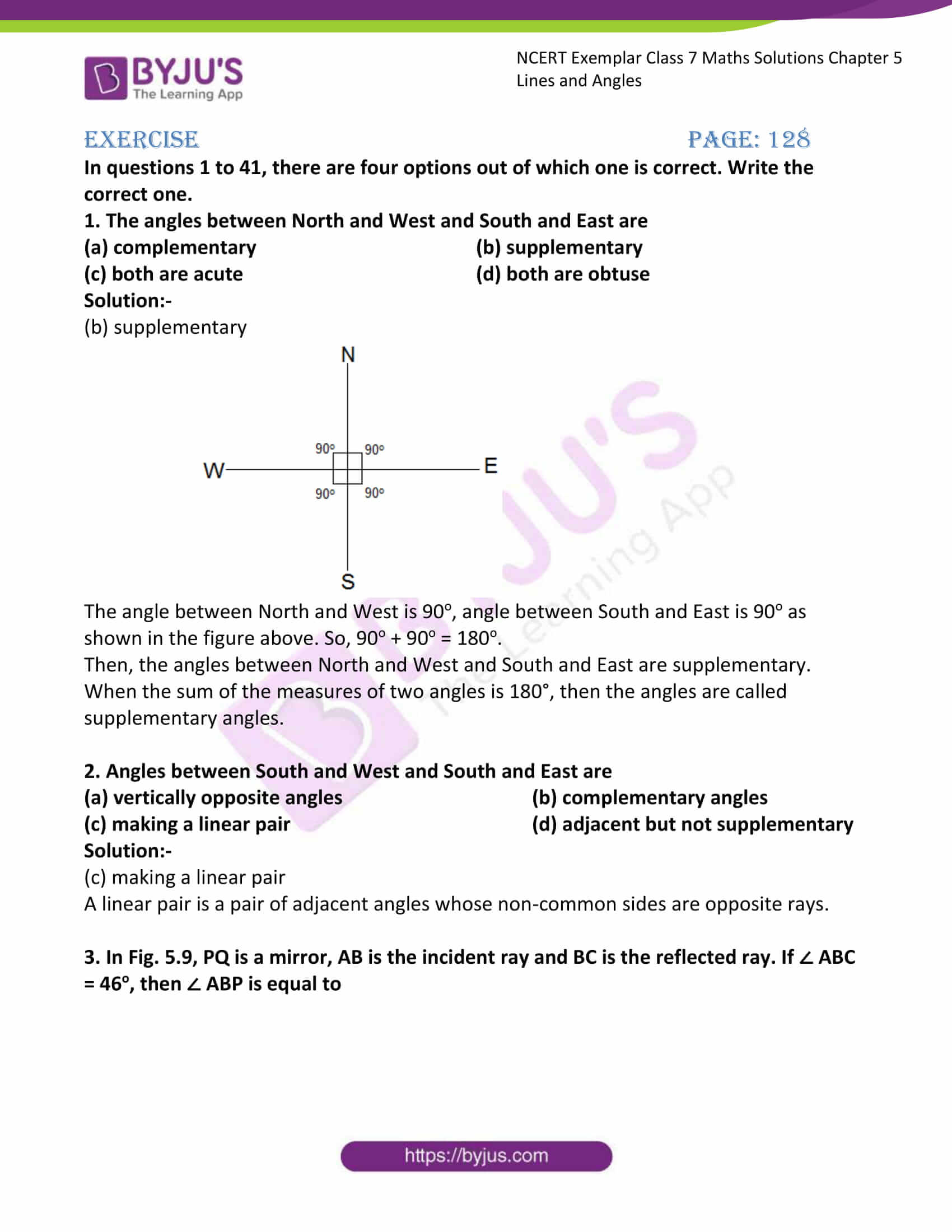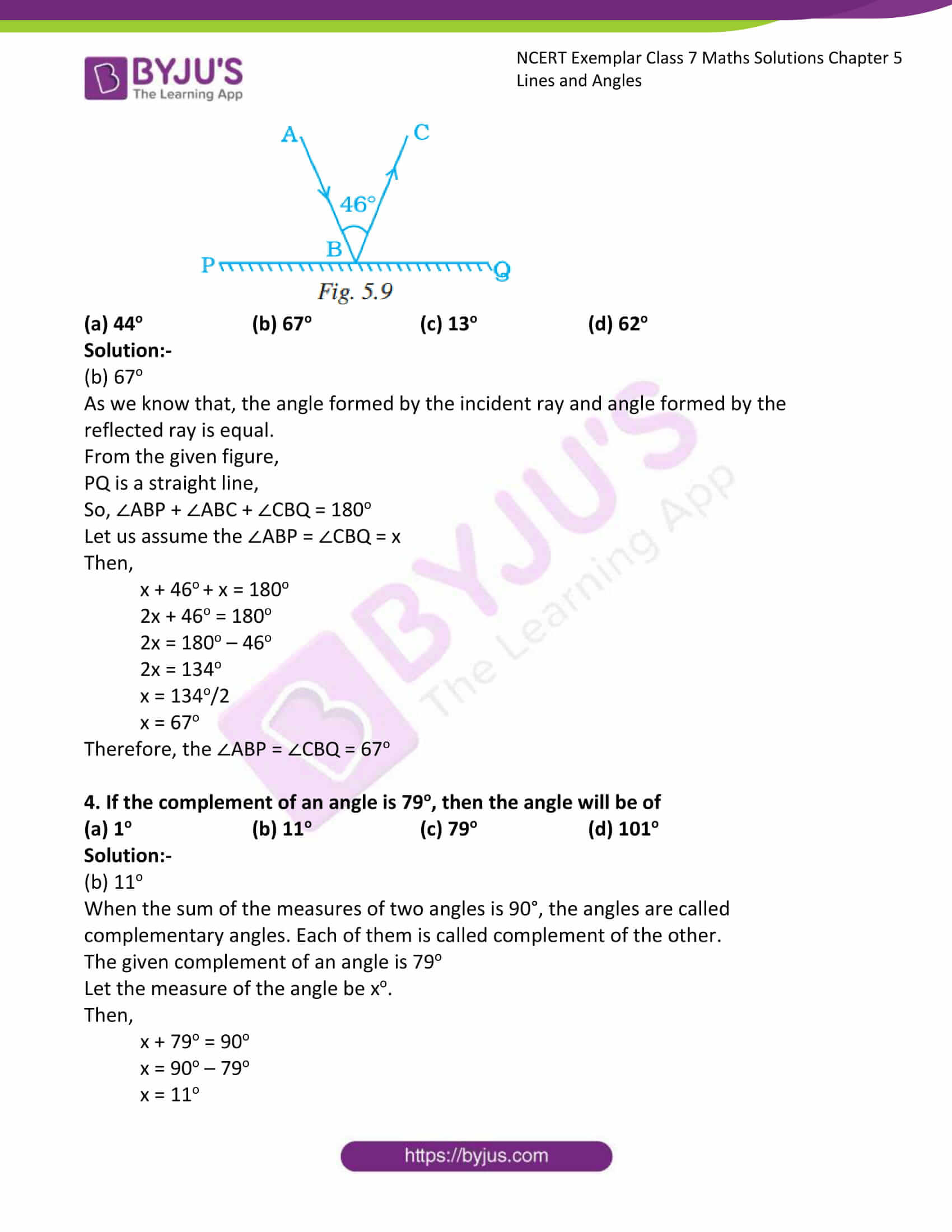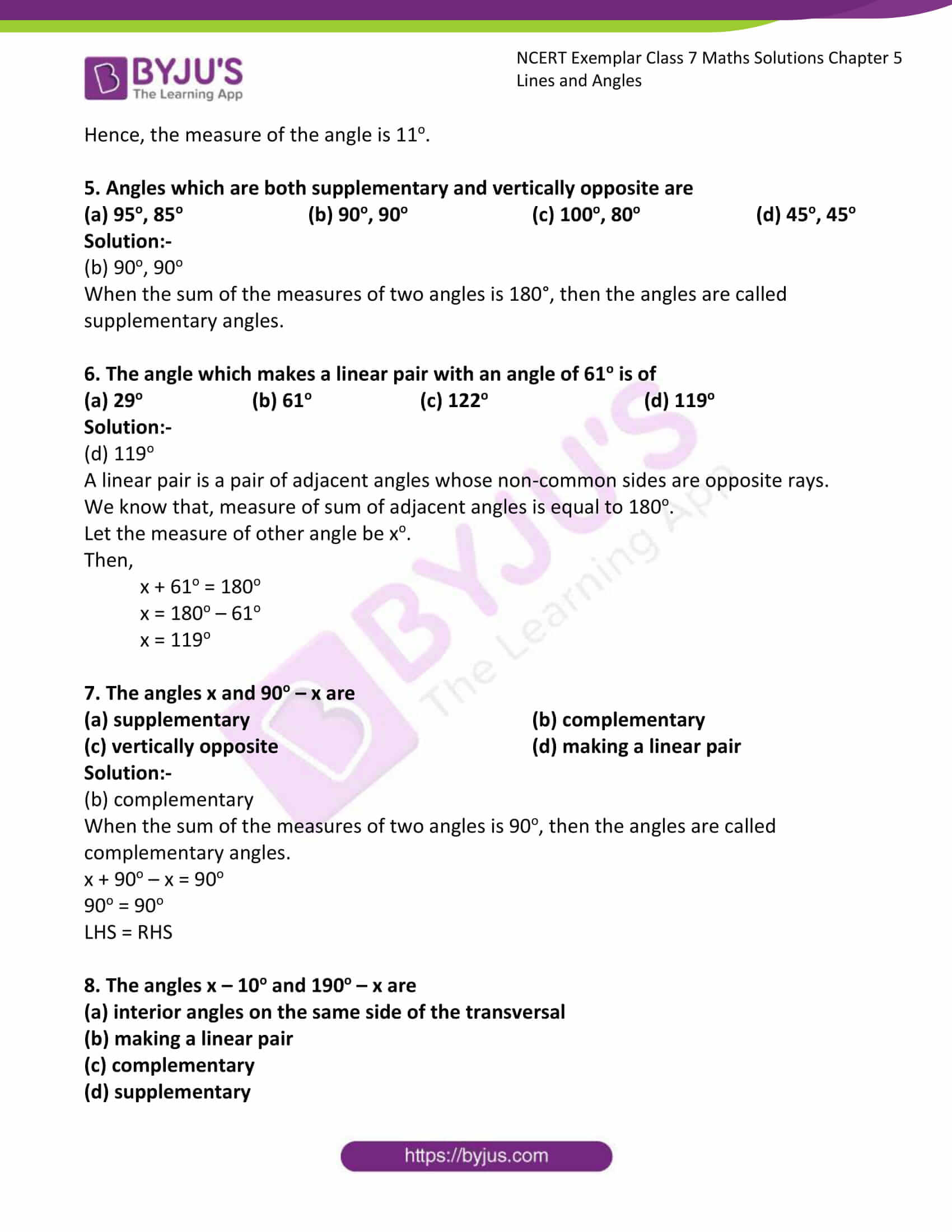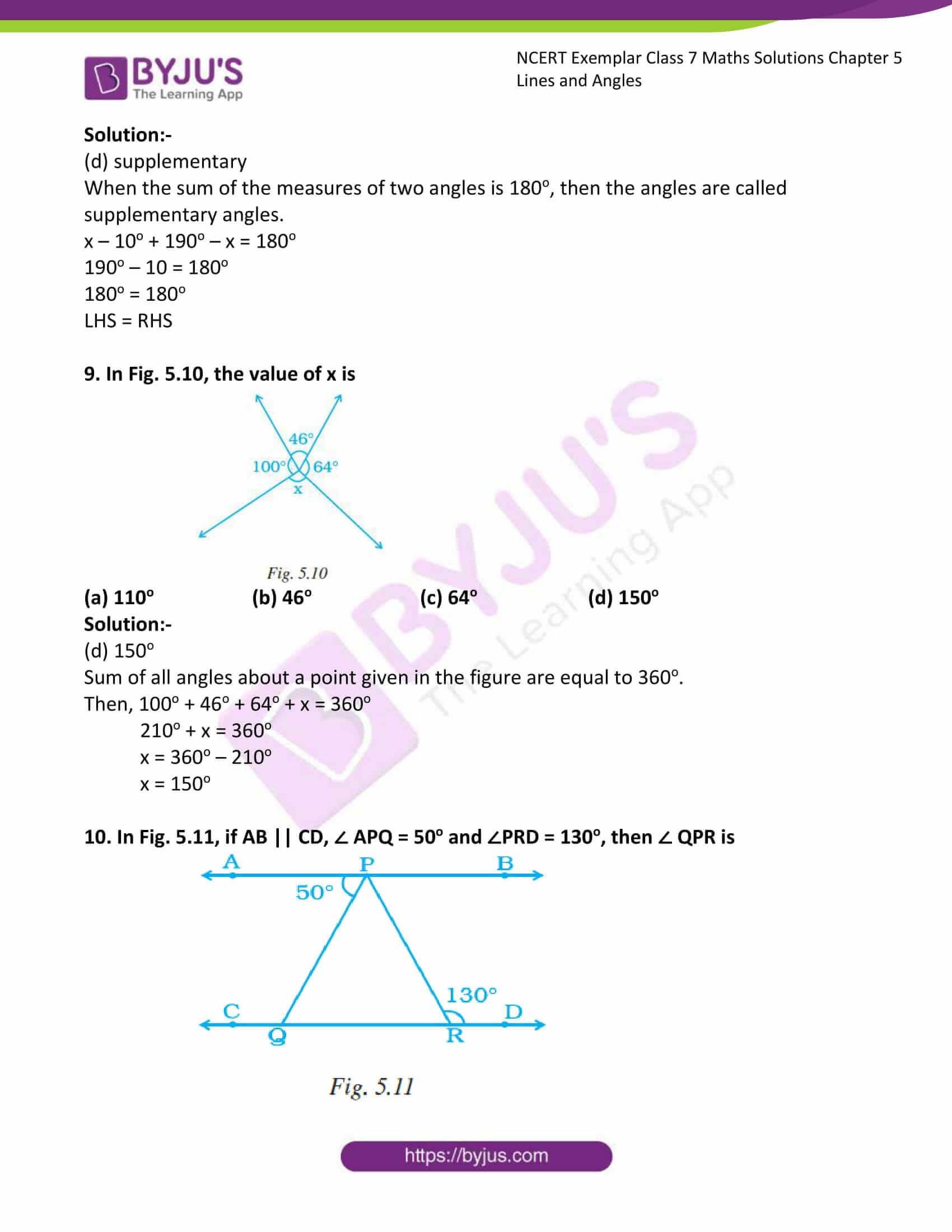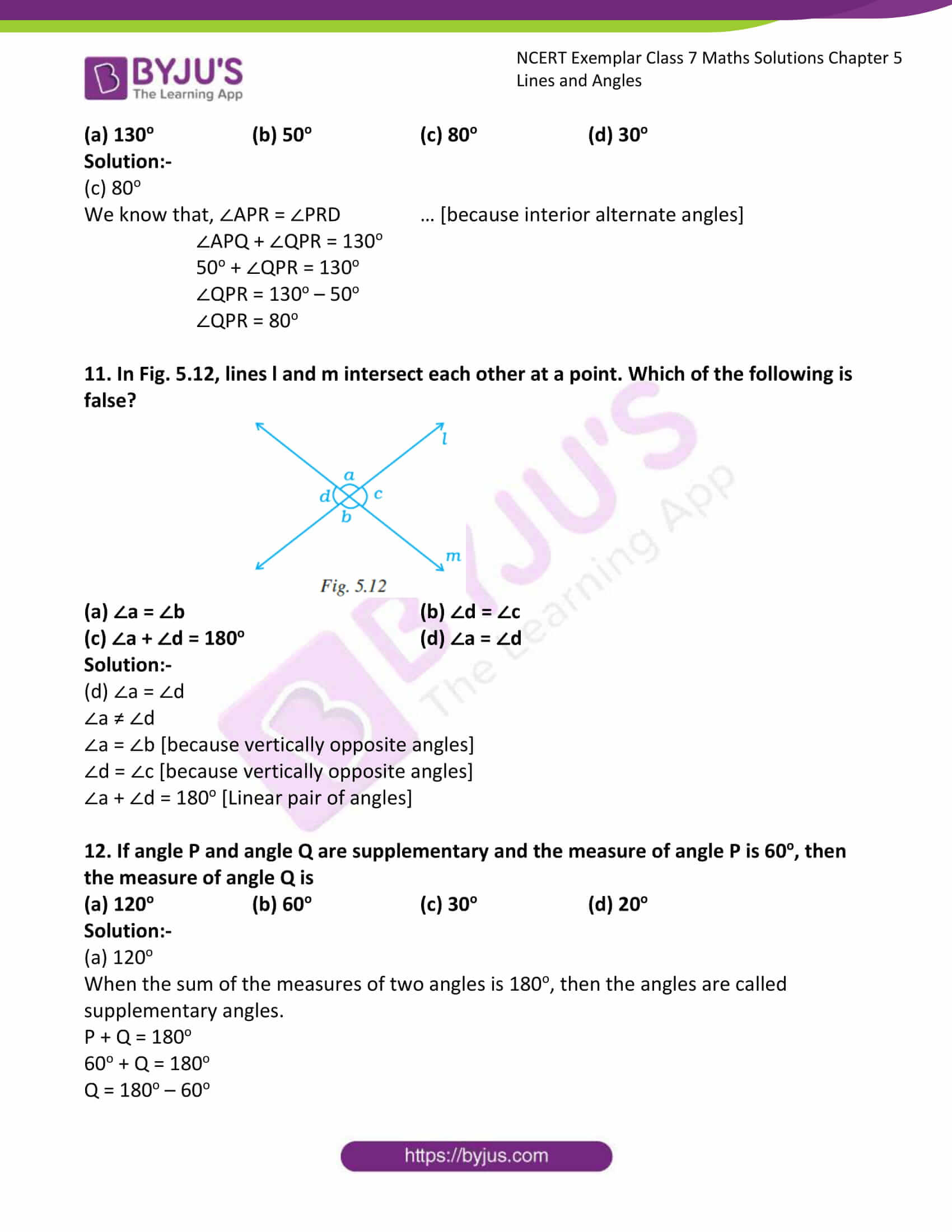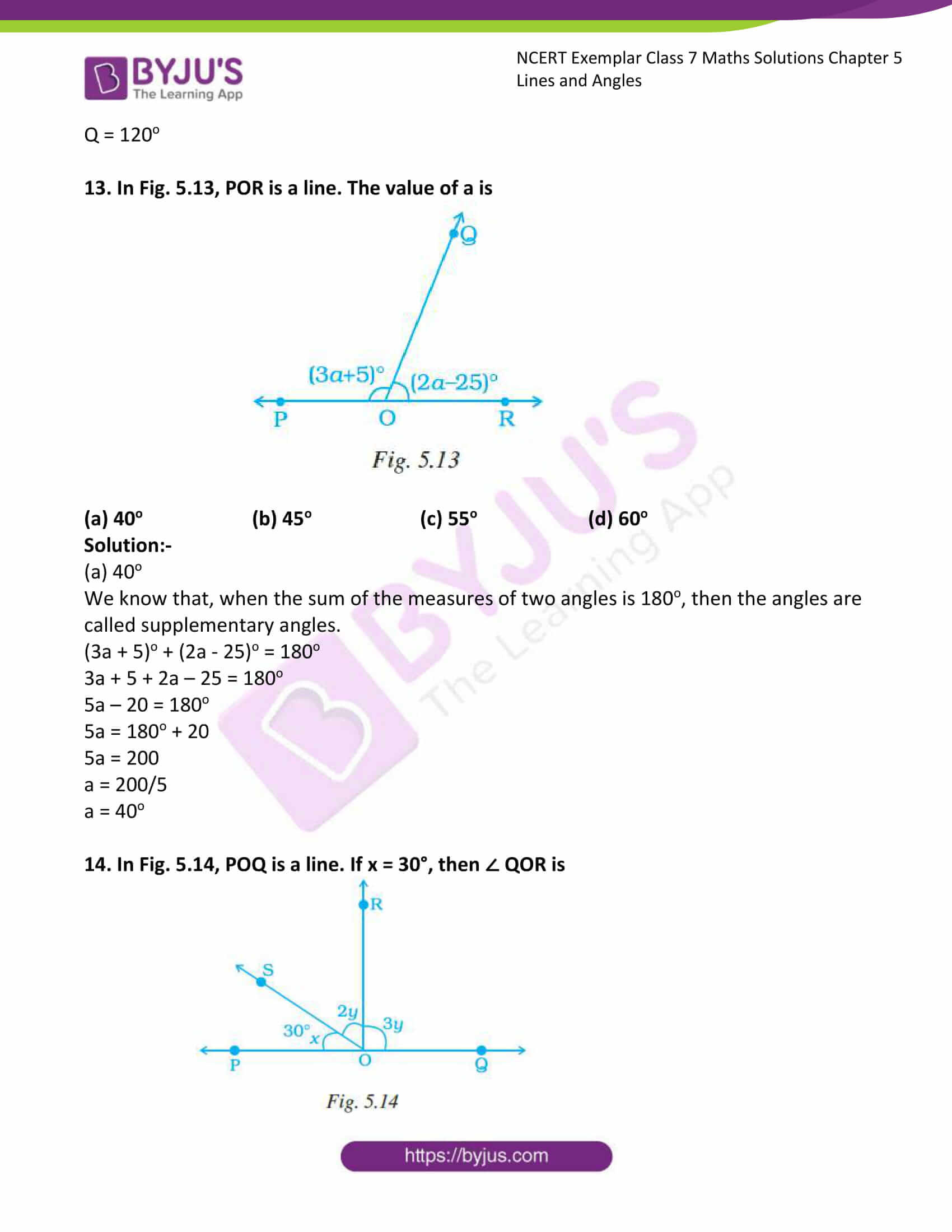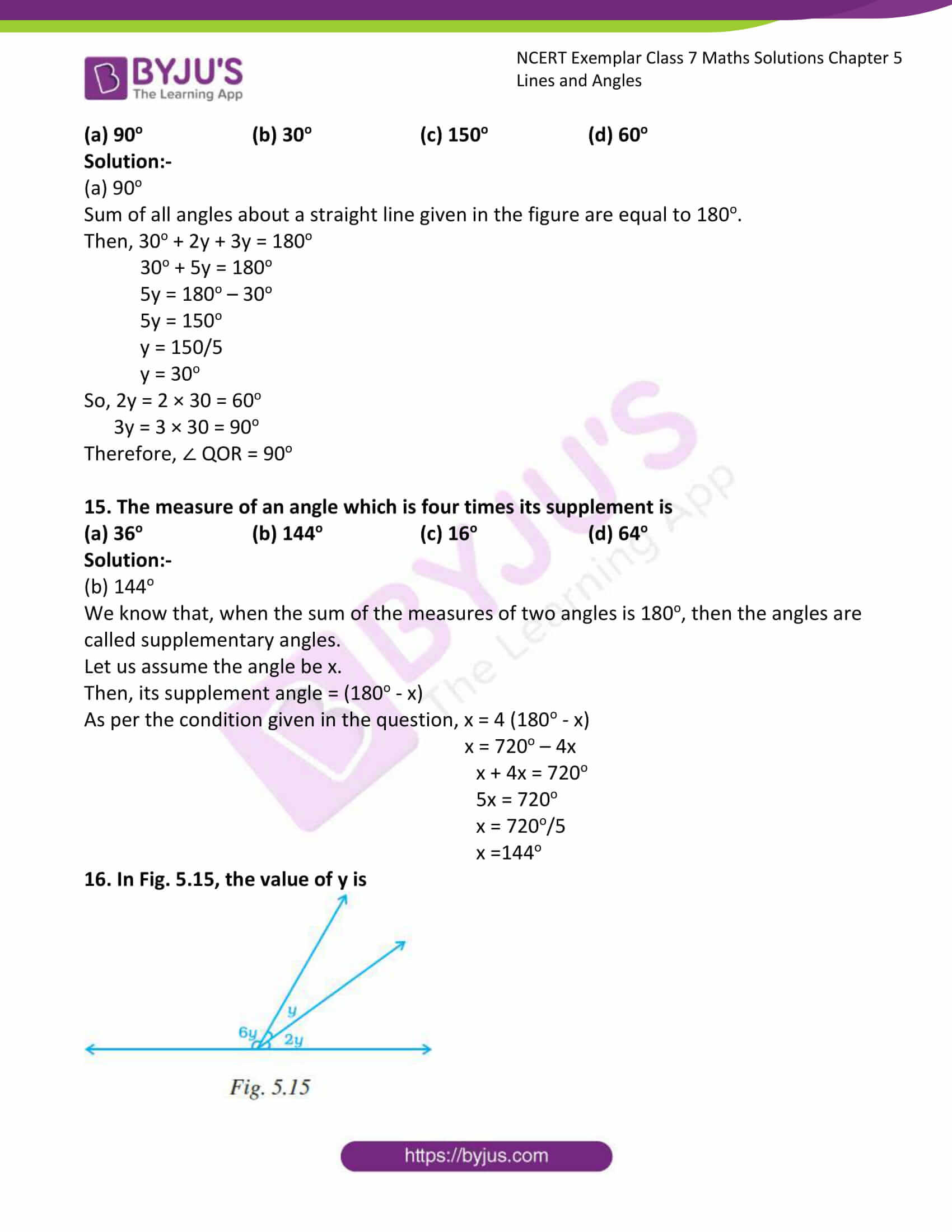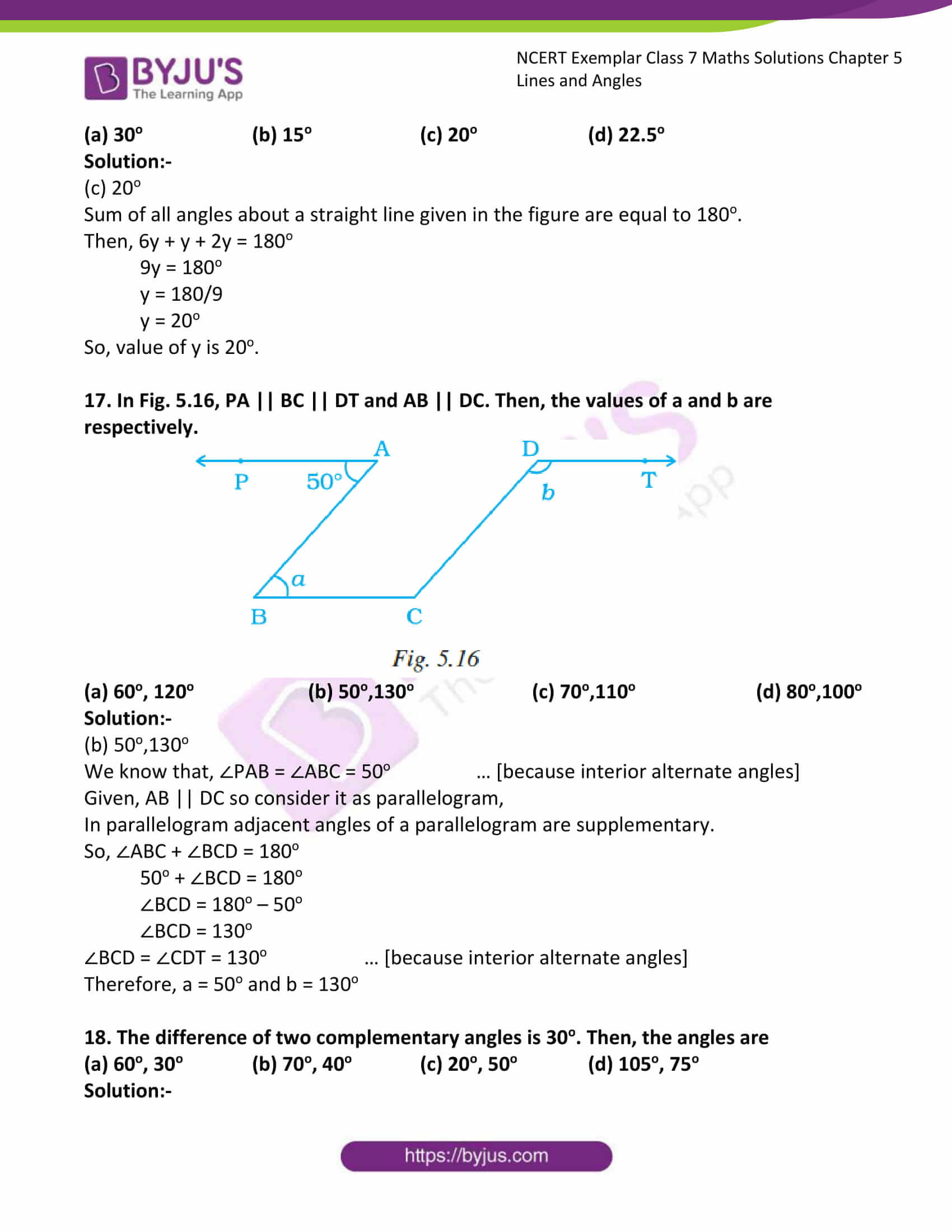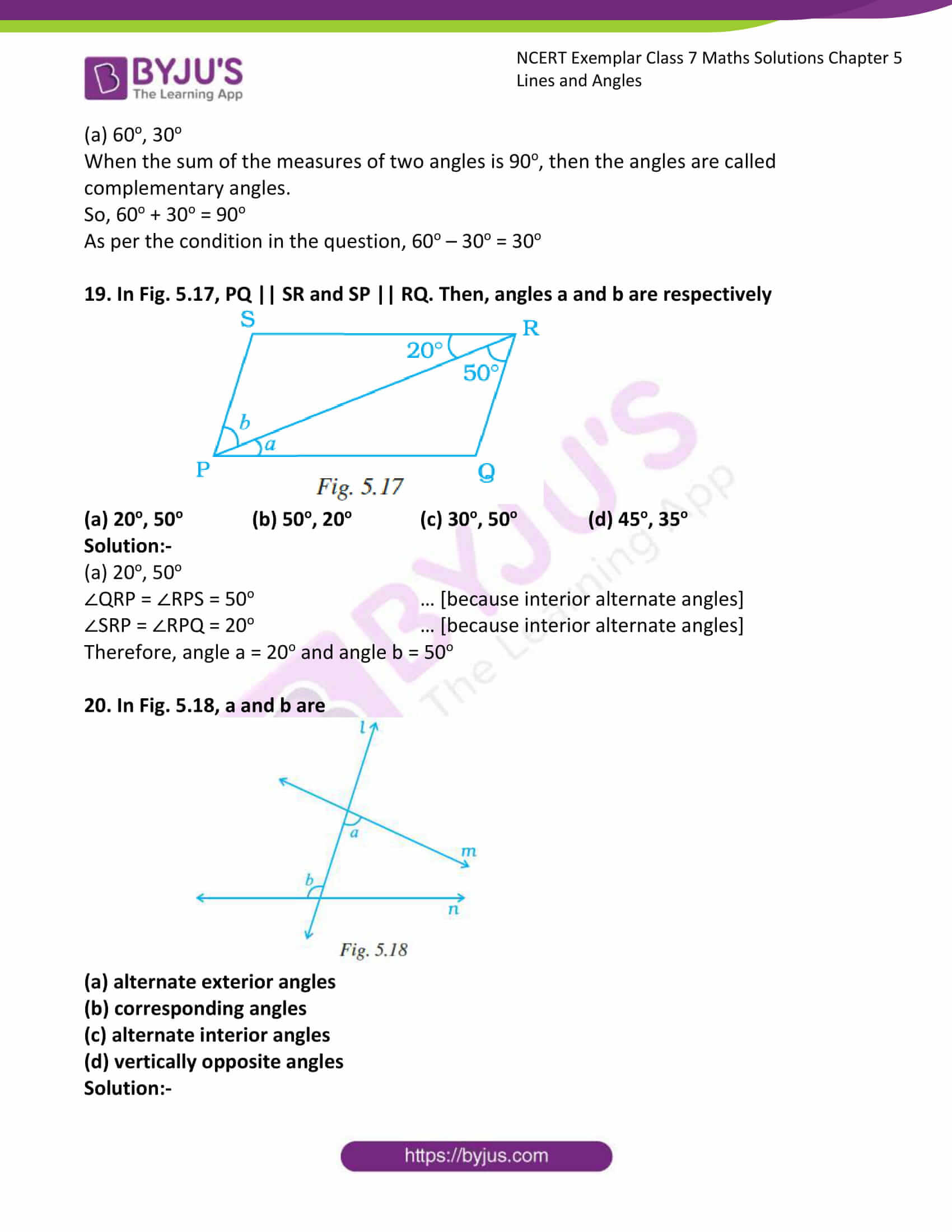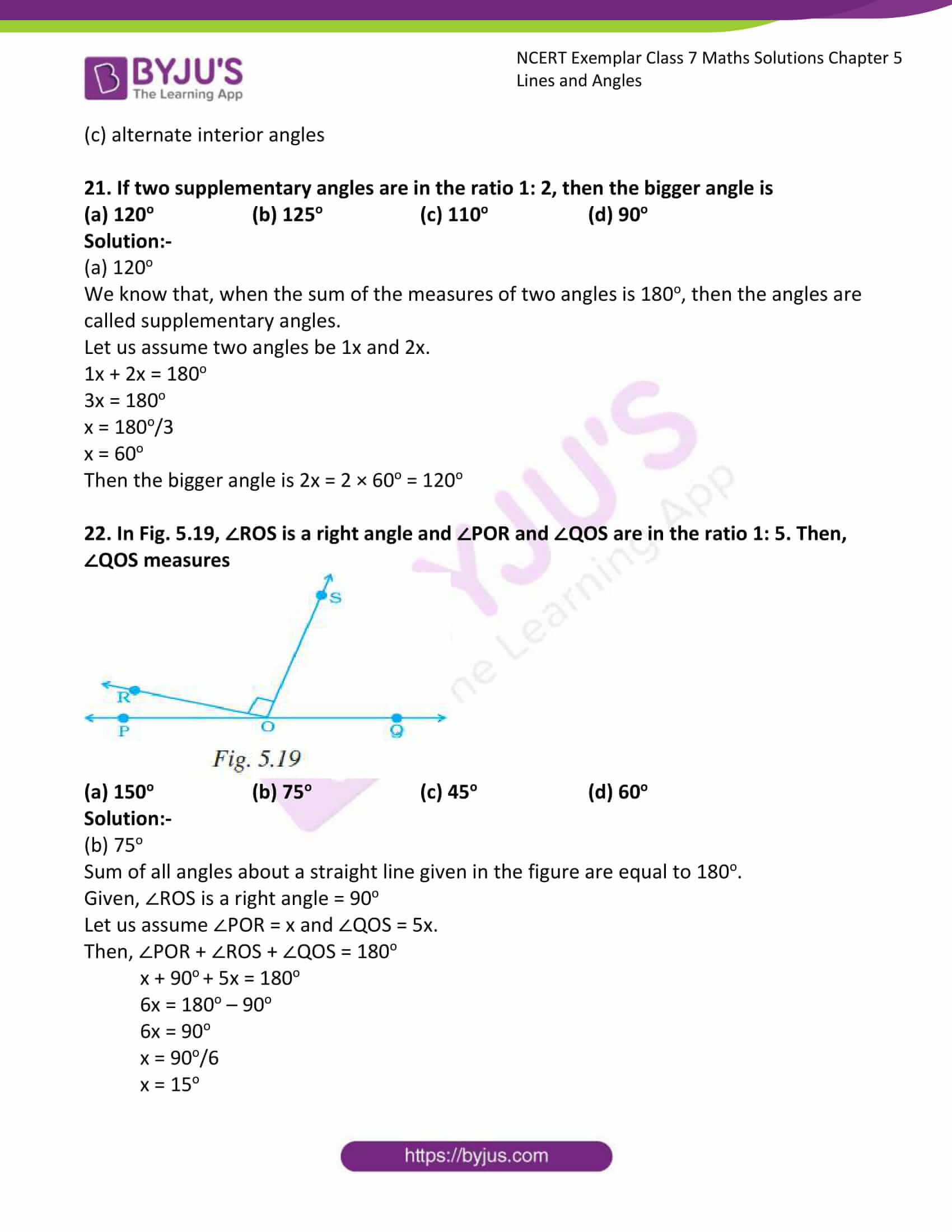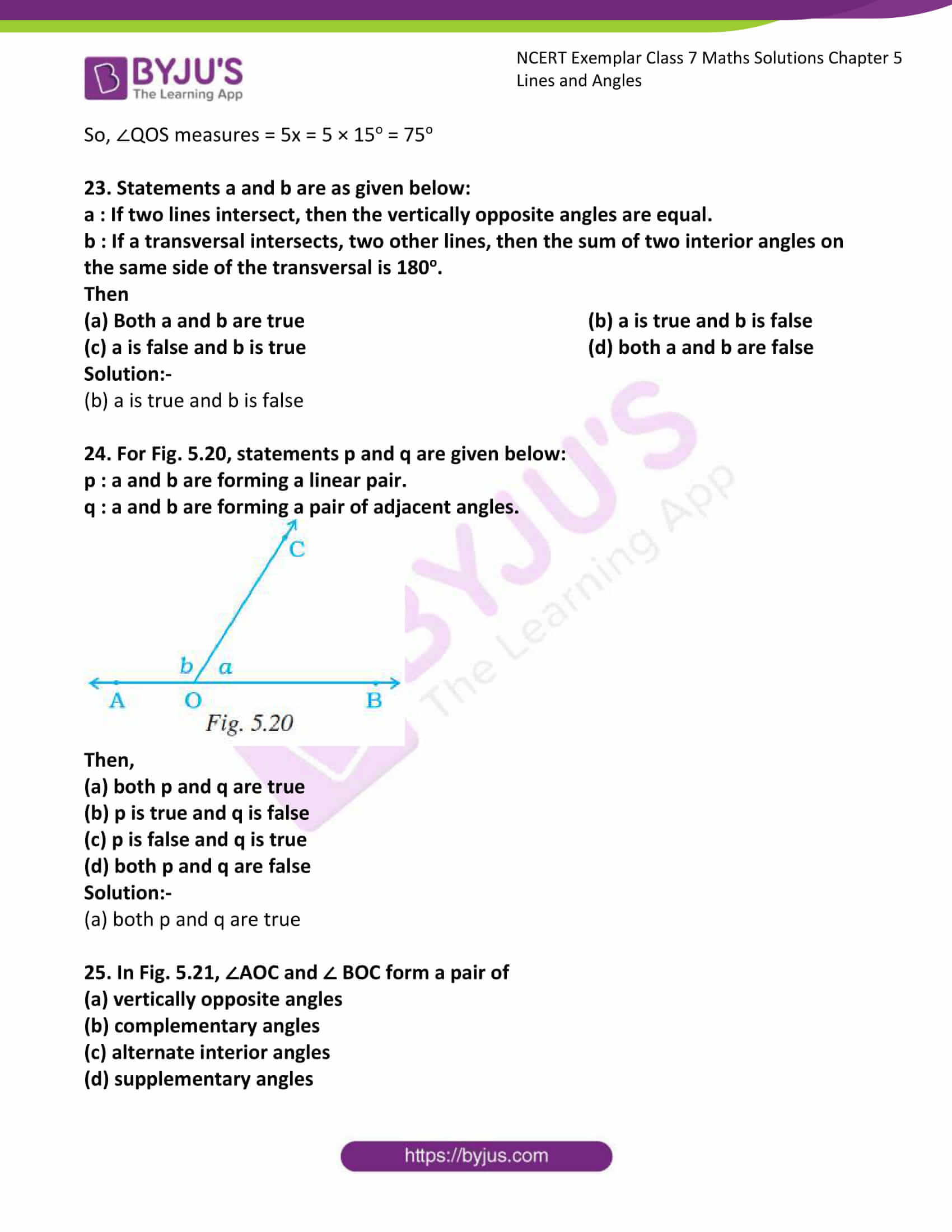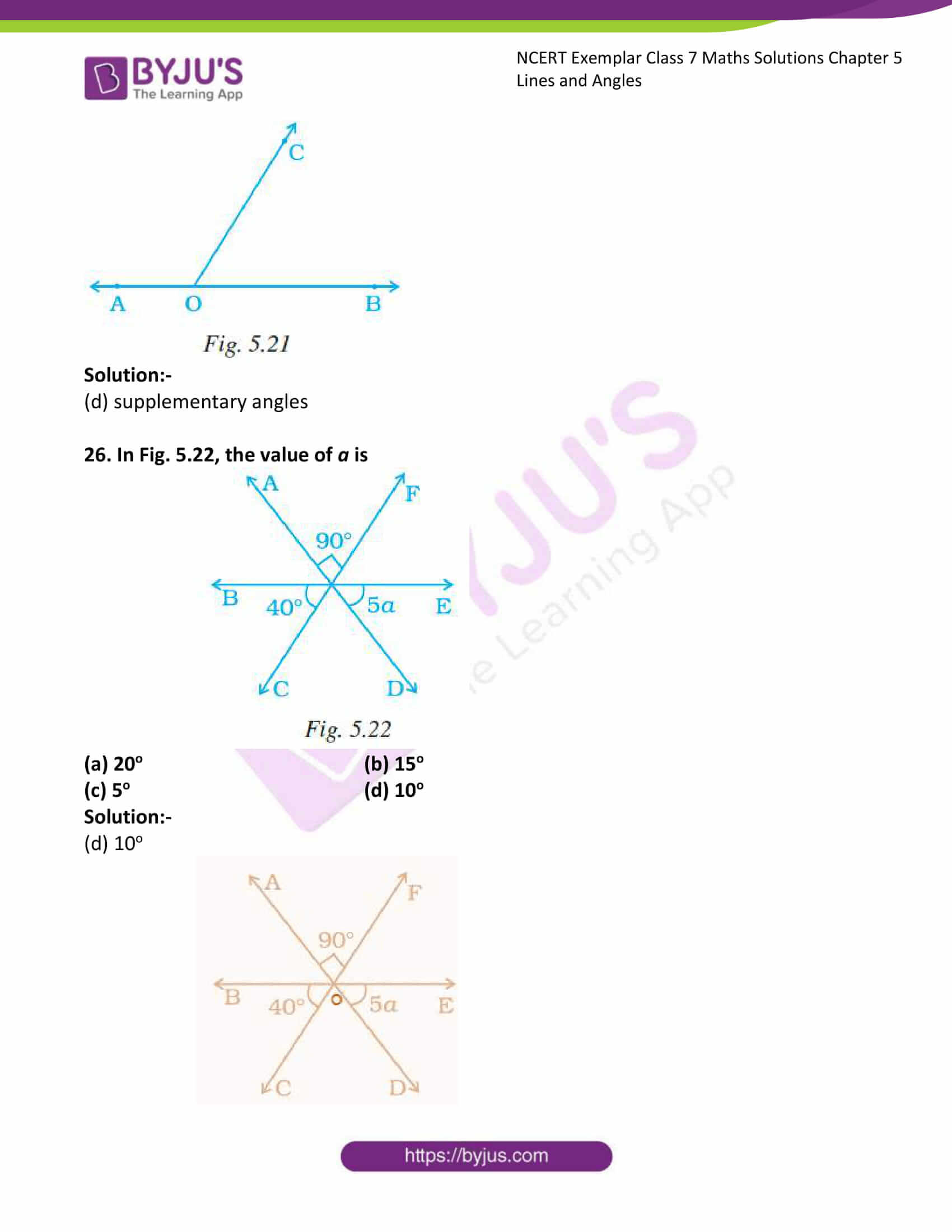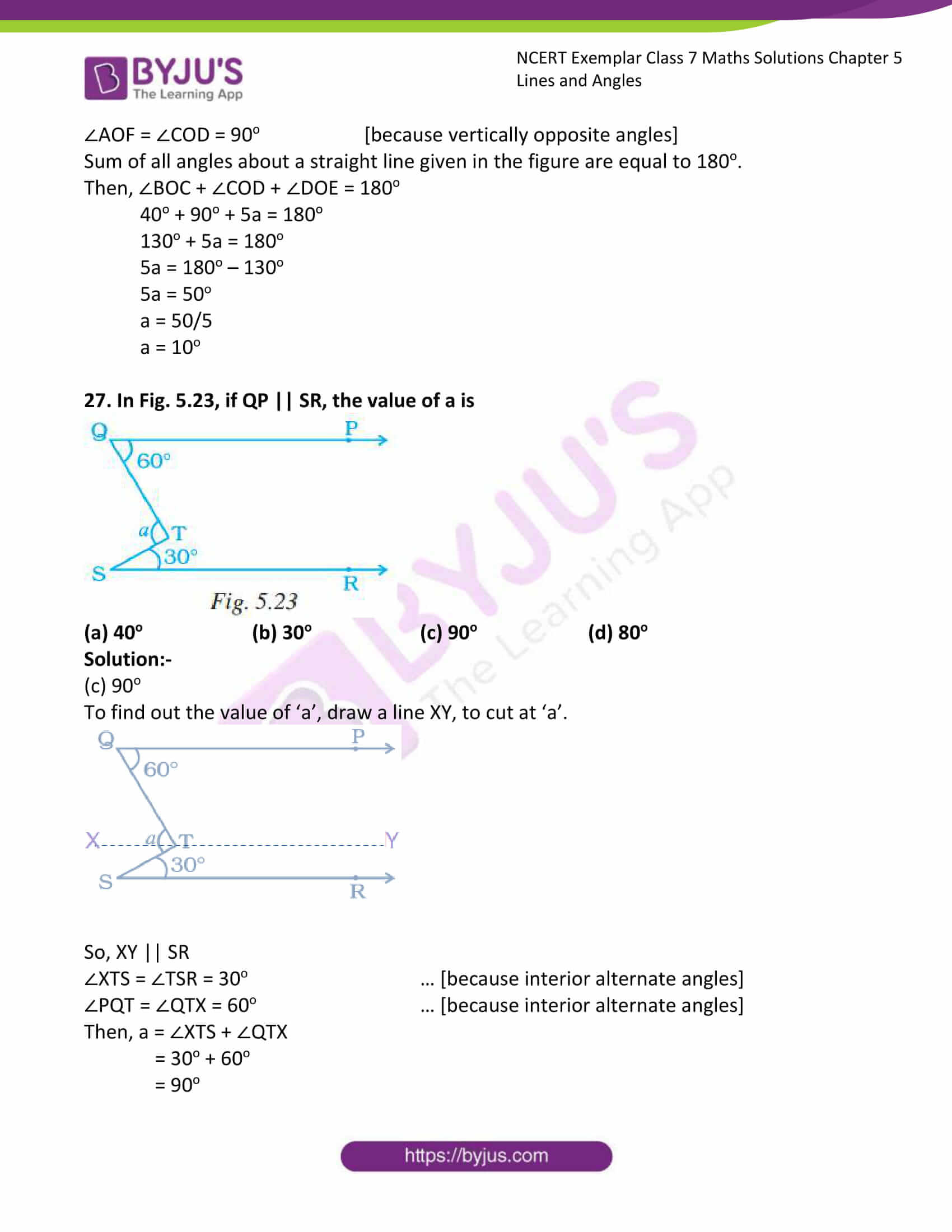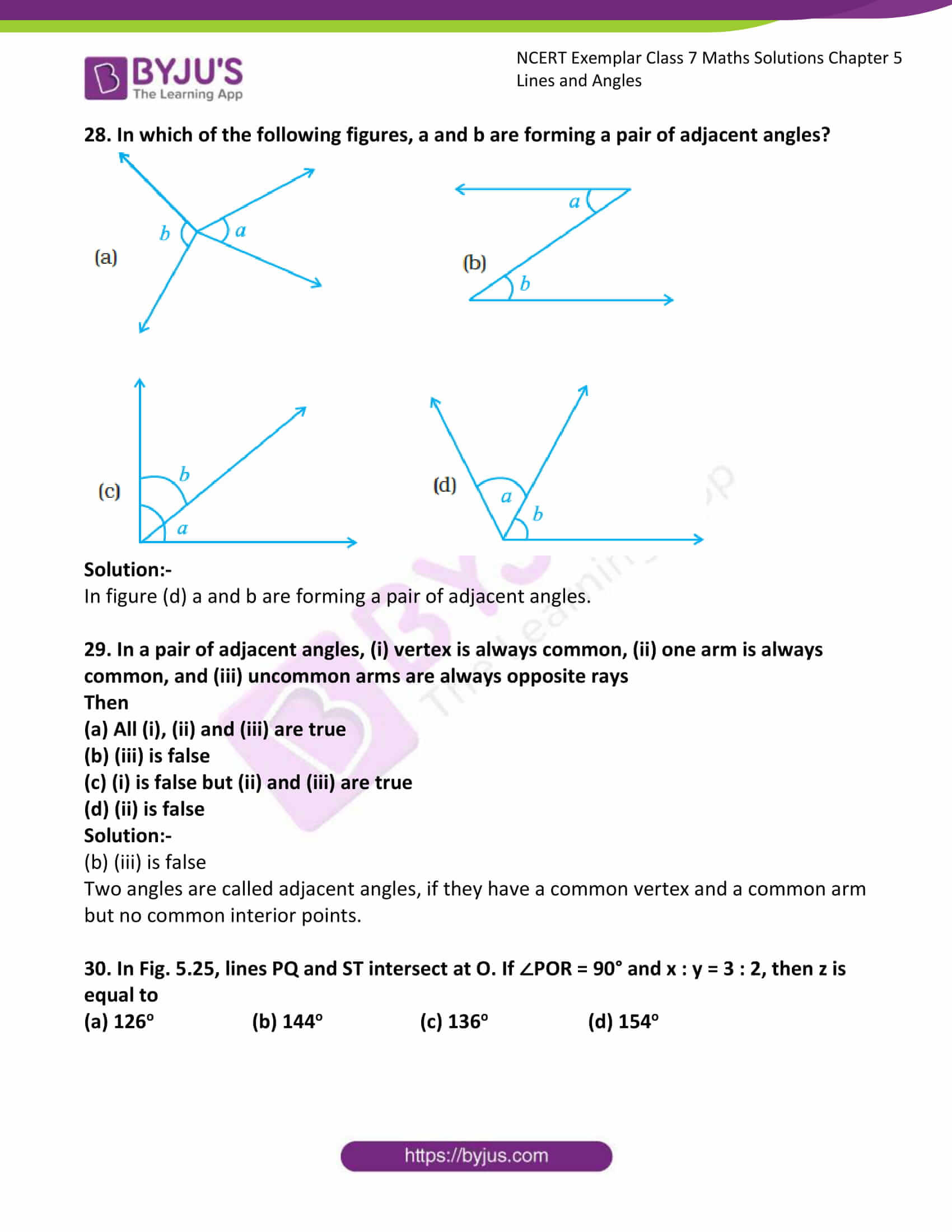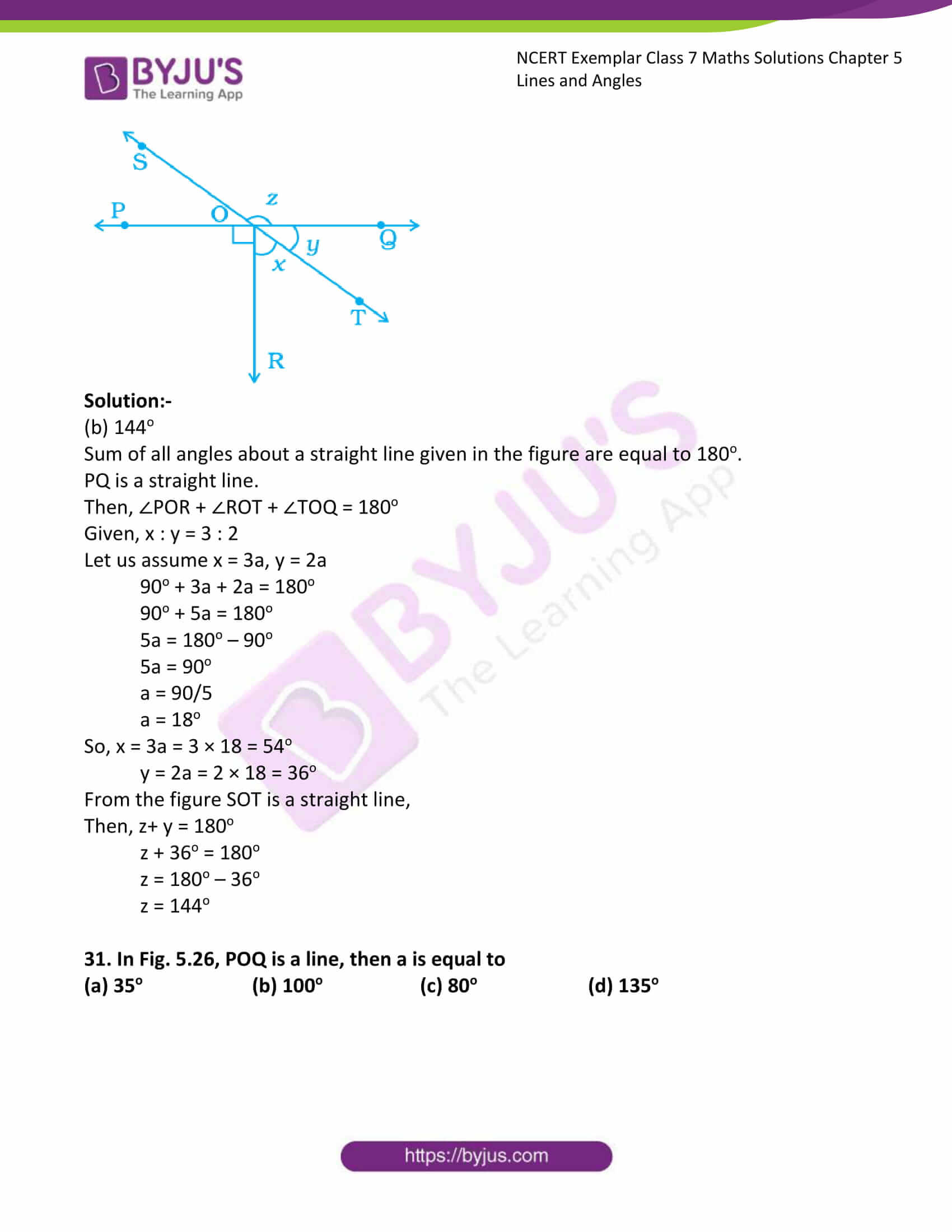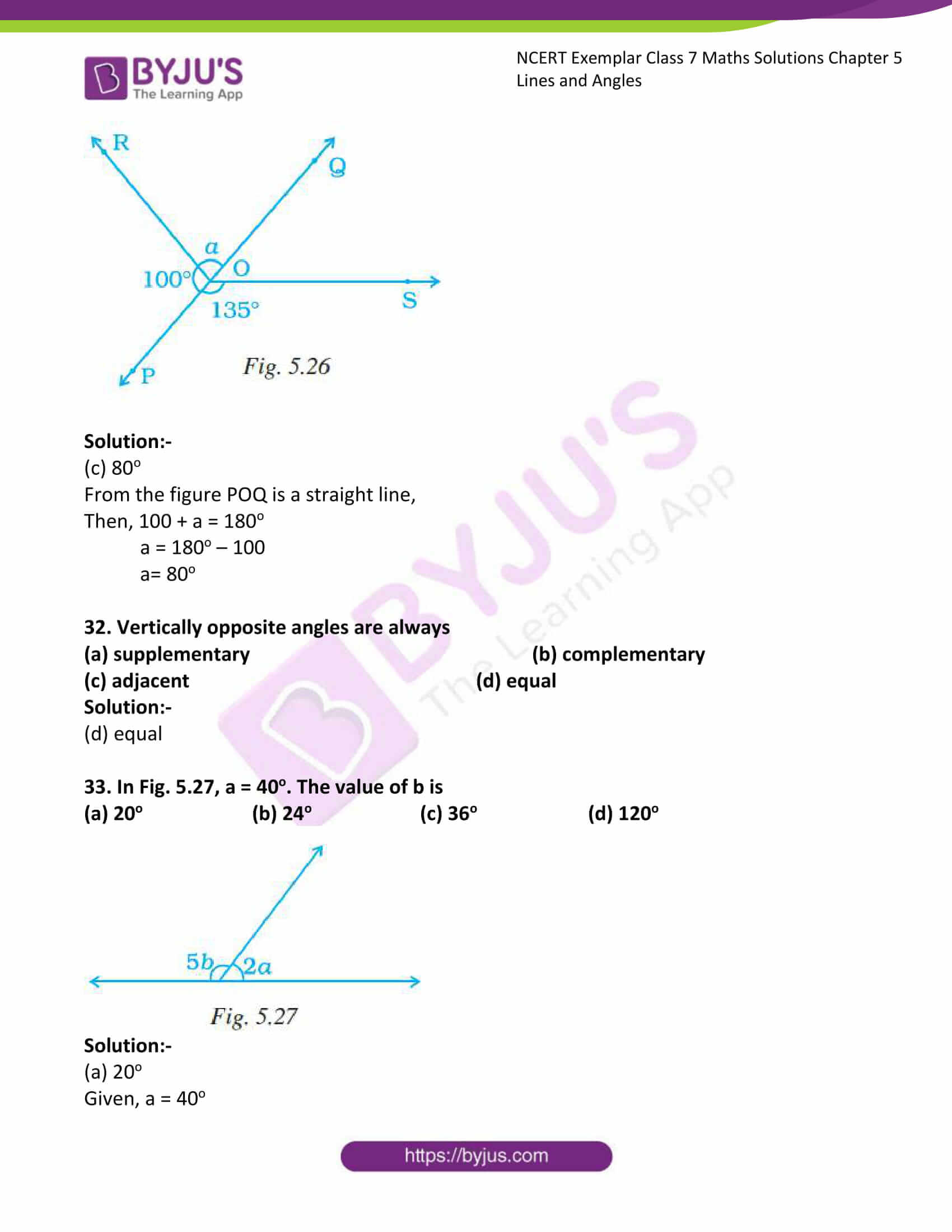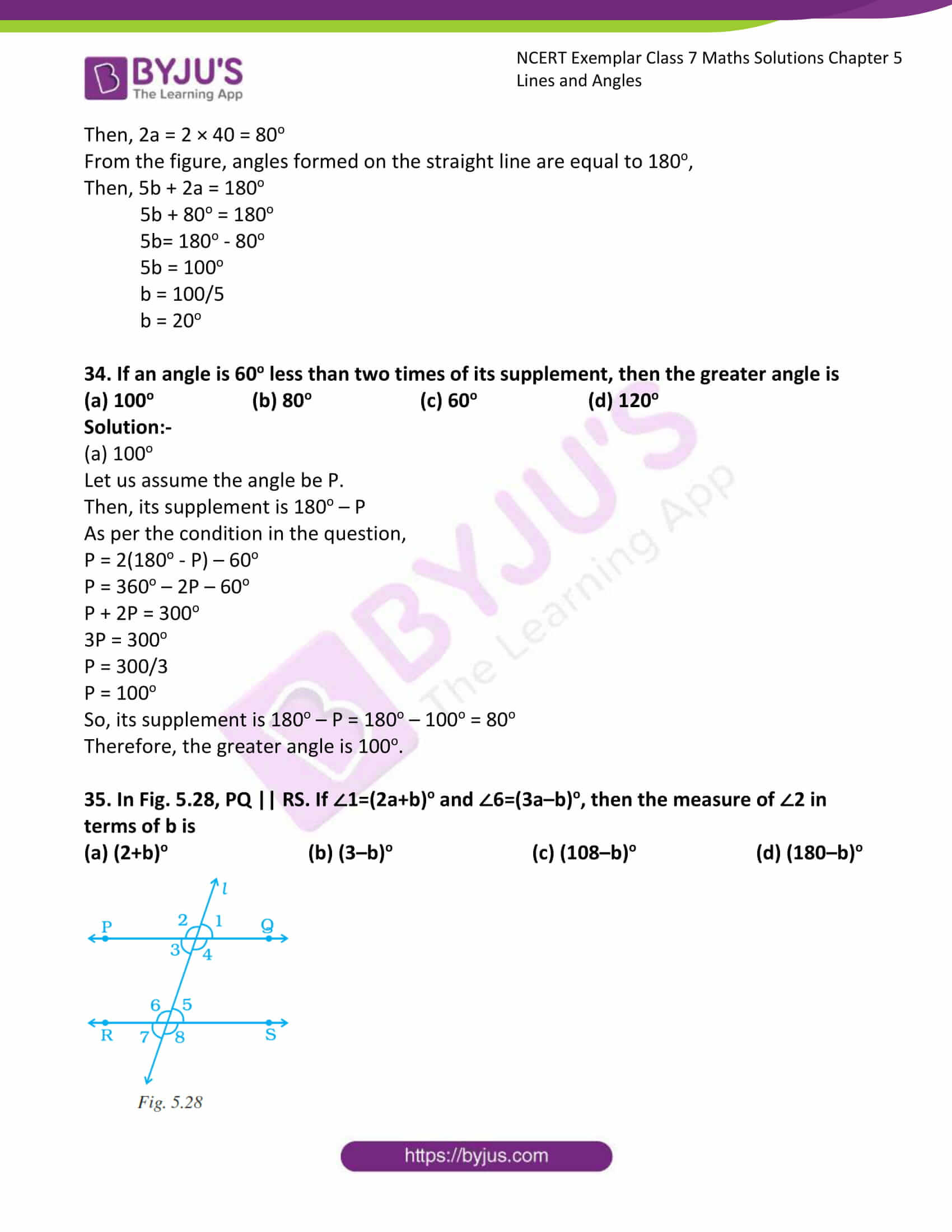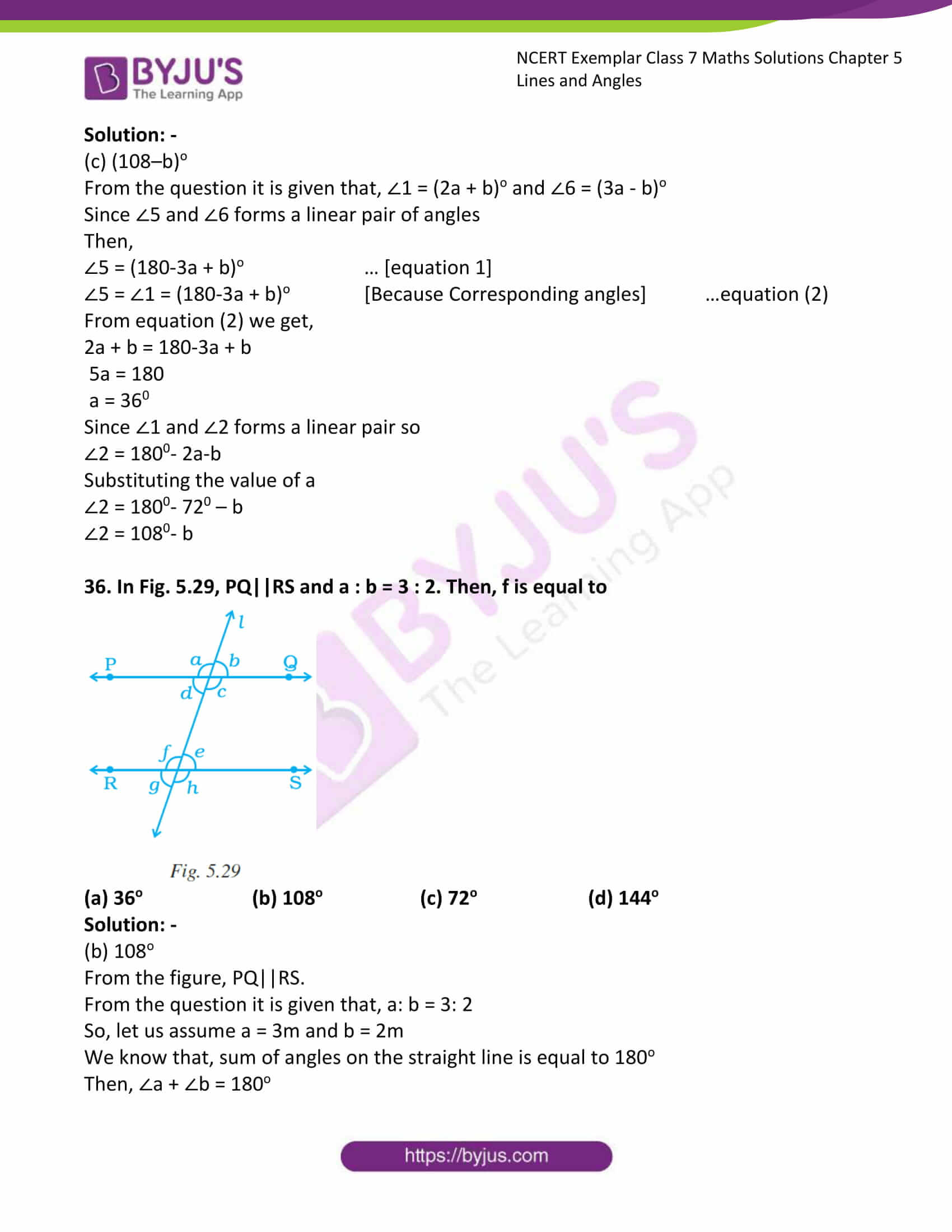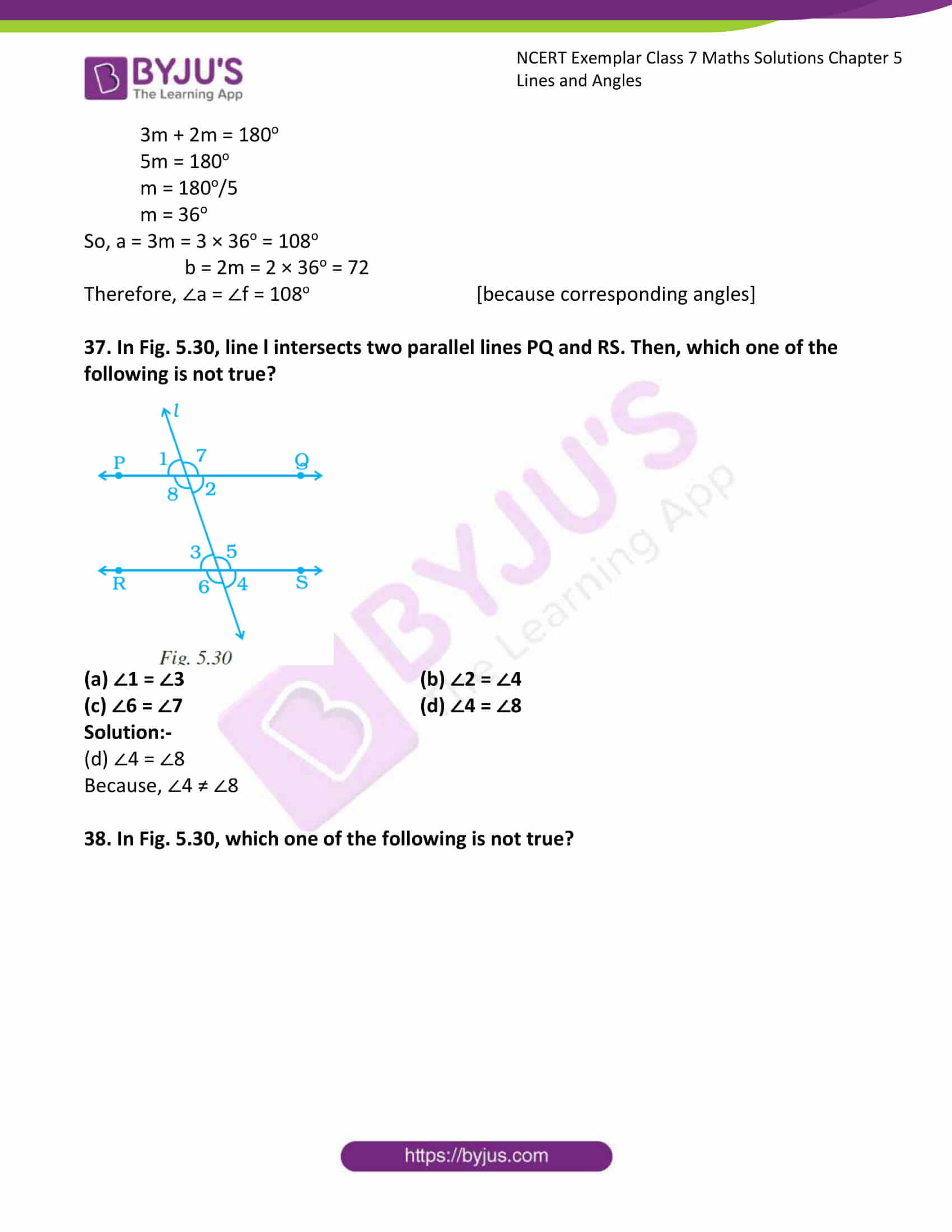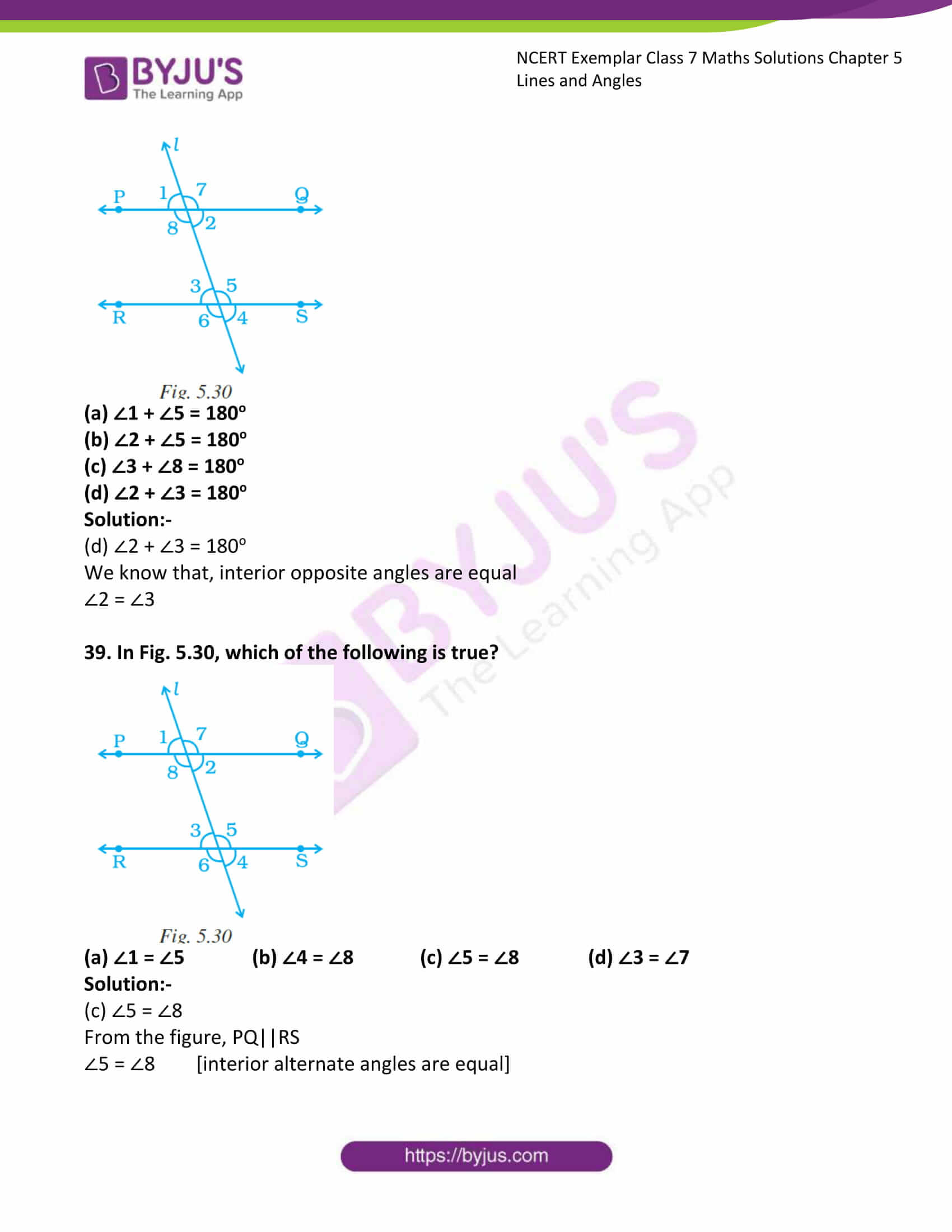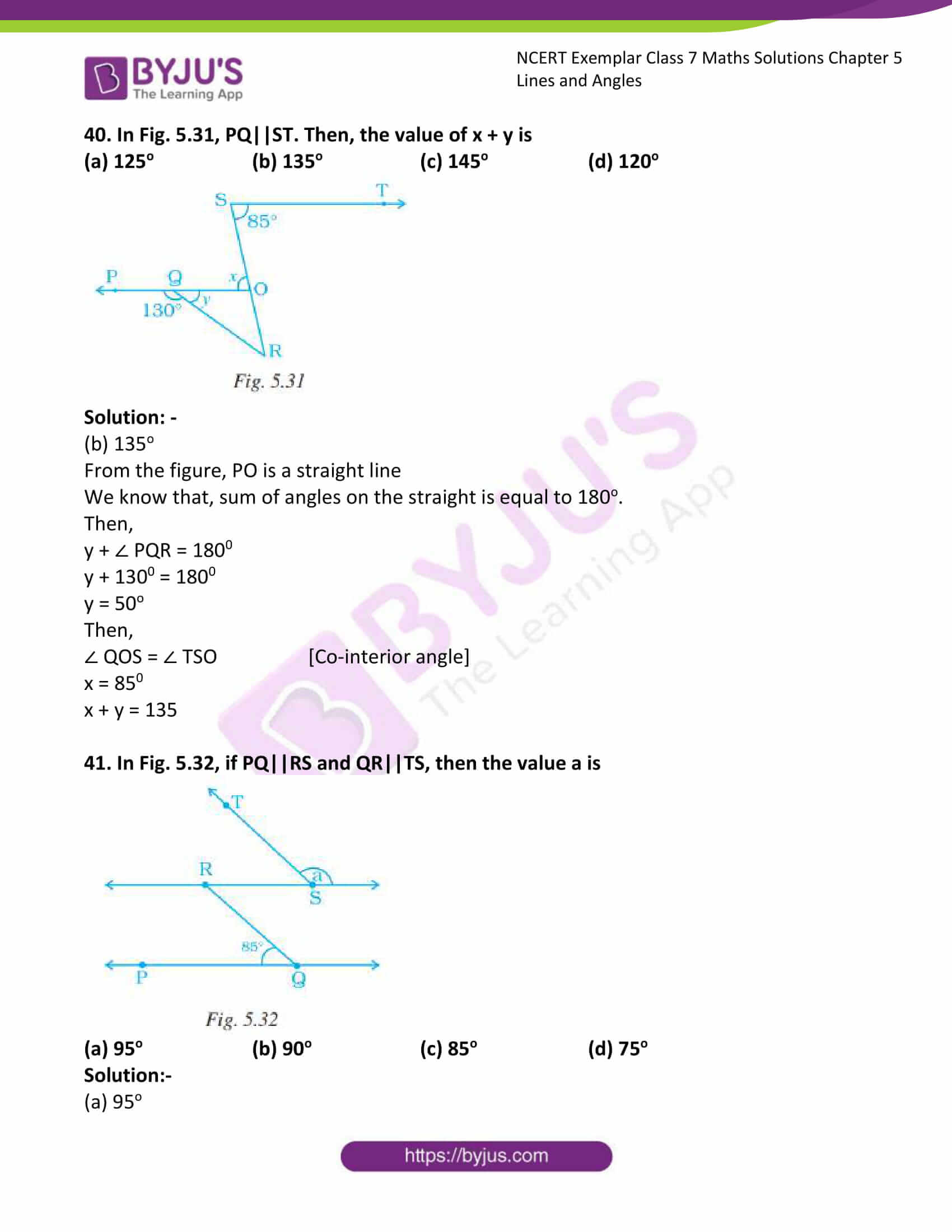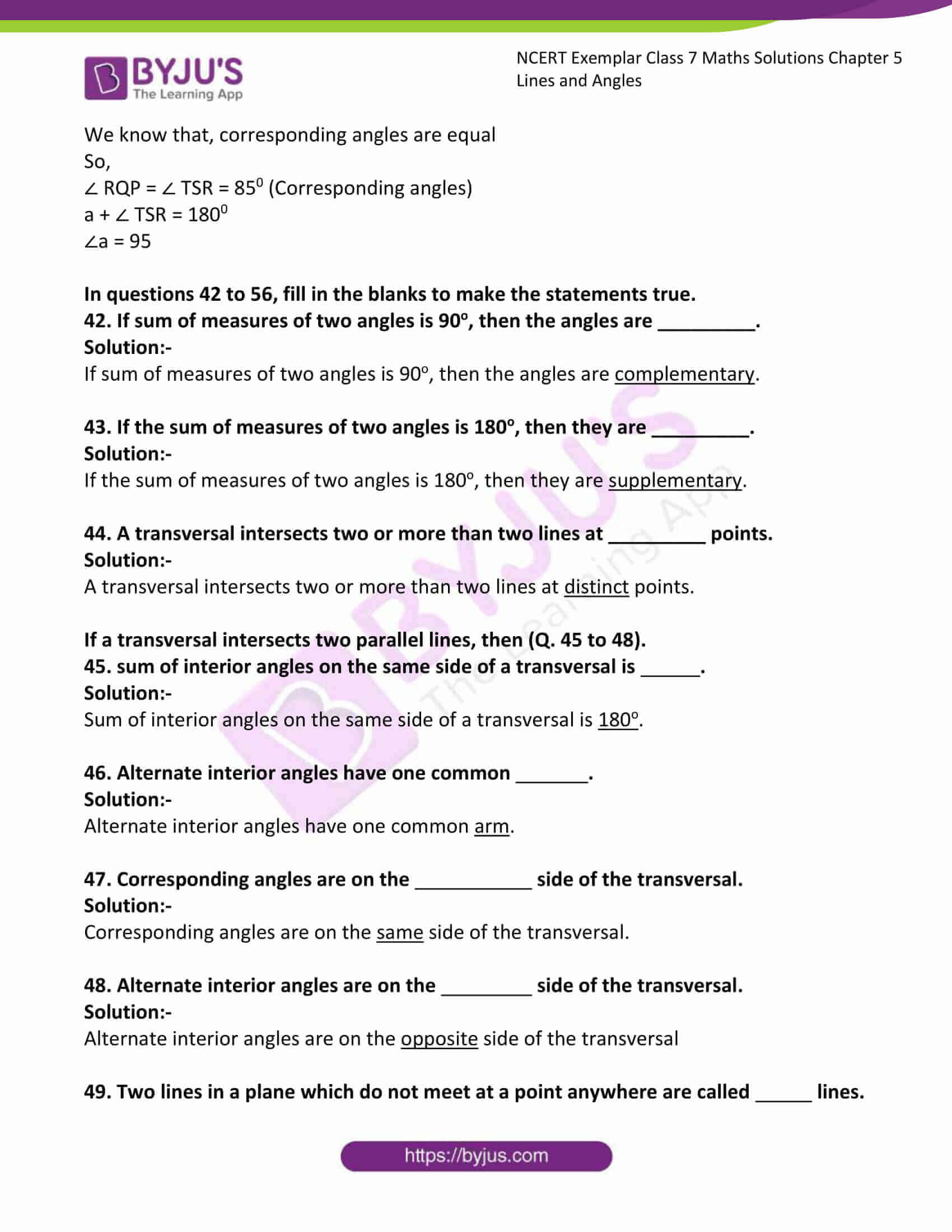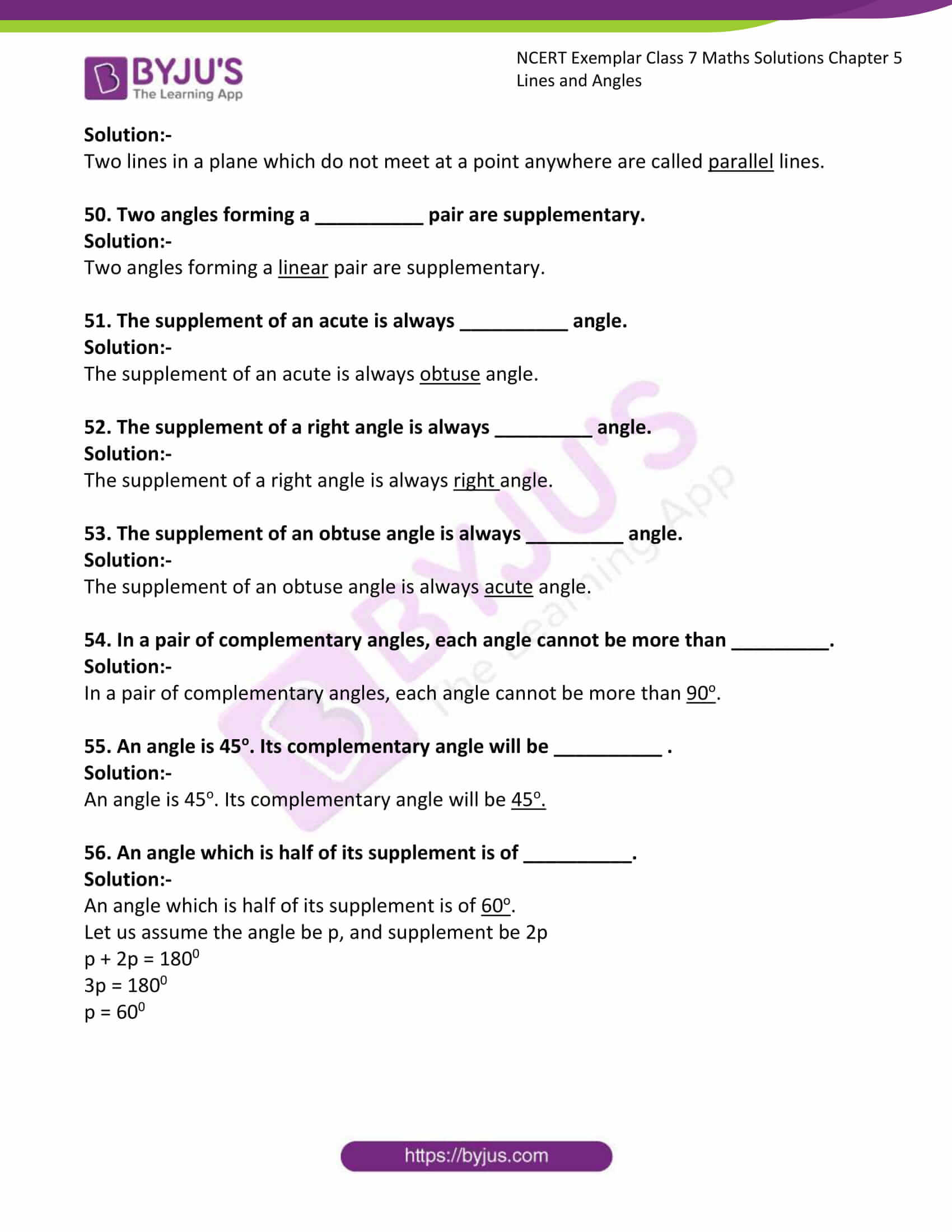### Access Answers to Maths NCERT Exemplar Solutions for Class 7 Chapter 5 Lines and Angles

Exercise Page: 128

In questions 1 to 41, there are four options, out of which one is correct. Write the correct one.

1. The angles between North and West and South and East are

(a) complementary (b) supplementary

(c) both are acute (d) both are obtuse

Solution:-

(b) supplementary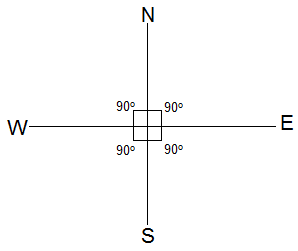The angle between North and West is 90o, the angle between South and East is 90o, as shown in the figure above. So, 90o + 90o = 180o.

Then, the angles between North and West and South and East are supplementary.

When the sum of the measures of two angles is 180°, then the angles are called supplementary angles.

2. Angles between South and West and South and East are

(a) vertically opposite angles (b) complementary angles

(c) making a linear pair (d) adjacent but not supplementary

Solution:-

(c) making a linear pair

A linear pair is a pair of adjacent angles whose non-common sides are opposite rays.

3. In Fig. 5.9, PQ is a mirror, AB is the incident ray and BC is the reflected ray. If ∠ ABC = 46o, then ∠ ABP is equal to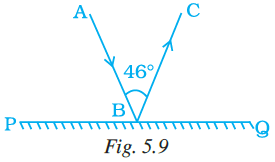(a) 44o (b) 67o (c) 13o (d) 62o

Solution:-

(b) 67o

As we know that, the angle formed by the incident ray and angle formed by the reflected ray is equal.

From the given figure,

PQ is a straight line,

So, ∠ABP + ∠ABC + ∠CBQ = 180o

Let us assume the ∠ABP = ∠CBQ = x

Then,

x + 46o + x = 180o

2x + 46o = 180o

2x = 180o – 46o

2x = 134o

x = 134o/2

x = 67o

Therefore, the ∠ABP = ∠CBQ = 67o

4. If the complement of an angle is 79o, then the angle will be of

(a) 1o (b) 11o (c) 79o (d) 101o

Solution:-

(b) 11o

When the sum of the measures of two angles is 90°, the angles are called complementary angles. Each of them is called complement of the other.

The given complement of an angle is 79o

Let the measure of the angle be xo.

Then,

x + 79o = 90o

x = 90o – 79o

x = 11o

Hence, the measure of the angle is 11o.

5. Angles which are both supplementary and vertically opposite are

(a) 95o, 85o (b) 90o, 90o (c) 100o, 80o (d) 45o, 45o

Solution:-

(b) 90o, 90o

When the sum of the measures of two angles is 180°, then the angles are called supplementary angles.

6. The angle which makes a linear pair with an angle of 61o is of

(a) 29o (b) 61o (c) 122o (d) 119o

Solution:-

(d) 119o

A linear pair is a pair of adjacent angles whose non-common sides are opposite rays.

We know that, measure of sum of adjacent angles is equal to 180o.

Let the measure of other angle be xo.

Then,

x + 61o = 180o

x = 180o – 61o

x = 119o

7. The angles x and 90o – x are

(a) supplementary (b) complementary

(c) vertically opposite (d) making a linear pair

Solution:-

(b) complementary

When the sum of the measures of two angles is 90o, then the angles are called complementary angles.

x + 90o – x = 90o

90o = 90o

LHS = RHS

8. The angles x – 10o and 190o – x are

(a) interior angles on the same side of the transversal

(b) making a linear pair

(c) complementary

(d) supplementary

Solution:-

(d) supplementary

When the sum of the measures of two angles is 180o, then the angles are called supplementary angles.

x – 10o + 190o – x = 180o

190o – 10 = 180o

180o = 180o

LHS = RHS

9. In Fig. 5.10, the value of x is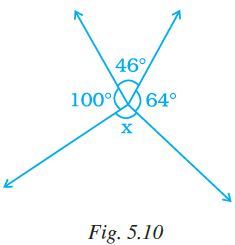(a) 110o (b) 46o (c) 64o (d) 150o

Solution:-

(d) 150o

The sum of all angles about a point given in the figure is equal to 360o.

Then, 100o + 46o + 64o + x = 360o

210o + x = 360o

x = 360o – 210o

x = 150o

10. In Fig. 5.11, if AB || CD, ∠ APQ = 50o and ∠PRD = 130o, then ∠ QPR is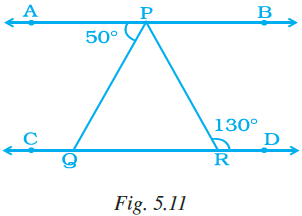(a) 130o (b) 50o (c) 80o (d) 30o

Solution:-

(c) 80o

We know that, ∠APR = ∠PRD … [because interior alternate angles]

∠APQ + ∠QPR = 130o

50o + ∠QPR = 130o

∠QPR = 130o – 50o

∠QPR = 80o

11. In Fig. 5.12, lines l and m intersect each other at a point. Which of the following is false?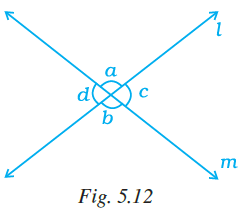(a) ∠a = ∠b (b) ∠d = ∠c

(c) ∠a + ∠d = 180o (d) ∠a = ∠d

Solution:-

(d) ∠a = ∠d

∠a ≠ ∠d

∠a = ∠b [because vertically opposite angles]

∠d = ∠c [because vertically opposite angles]

∠a + ∠d = 180o [Linear pair of angles]

12. If angle P and angle Q are supplementary and the measure of angle P is 60o, then the measure of angle Q is

(a) 120o (b) 60o (c) 30o (d) 20o

Solution:-

(a) 120o

When the sum of the measures of two angles is 180o, then the angles are called supplementary angles.

P + Q = 180o

60o + Q = 180o

Q = 180o – 60o

Q = 120o

13. In Fig. 5.13, POR is a line. The value of a is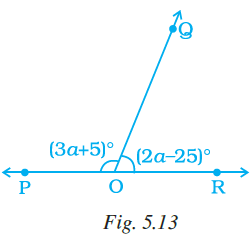(a) 40o (b) 45o (c) 55o (d) 60o

Solution:-

(a) 40o

We know that, when the sum of the measures of two angles is 180o, then the angles are called supplementary angles.

(3a + 5)o + (2a – 25)o = 180o

3a + 5 + 2a – 25 = 180o

5a – 20 = 180o

5a = 180o + 20

5a = 200

a = 200/5

a = 40o

14. In Fig. 5.14, POQ is a line. If x = 30°, then ∠ QOR is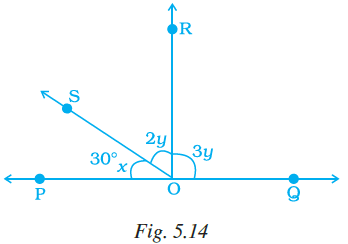(a) 90o (b) 30o (c) 150o (d) 60o

Solution:-

(a) 90o

Sum of all angles about a straight line given in the figure are equal to 180o.

Then, 30o + 2y + 3y = 180o

30o + 5y = 180o

5y = 180o – 30o

5y = 150o

y = 150/5

y = 30o

So, 2y = 2 × 30 = 60o

3y = 3 × 30 = 90o

Therefore, ∠ QOR = 90o

15. The measure of an angle which is four times its supplement is

(a) 36o (b) 144o (c) 16o (d) 64o

Solution:-

(b) 144o

We know that, when the sum of the measures of two angles is 180o, then the angles are called supplementary angles.

Let us assume the angle be x.

Then, its supplement angle = (180o – x)

As per the condition given in the question, x = 4 (180o – x)

x = 720o – 4x

x + 4x = 720o

5x = 720o

x = 720o/5

x =144o

16. In Fig. 5.15, the value of y is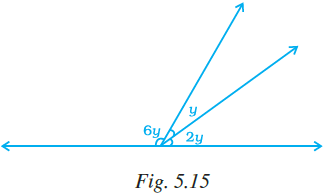(a) 30o (b) 15o (c) 20o (d) 22.5o

Solution:-

(c) 20o

The sum of all angles about a straight line given in the figure is equal to 180o.

Then, 6y + y + 2y = 180o

9y = 180o

y = 180/9

y = 20o

So, value of y is 20o.

17. In Fig. 5.16, PA || BC || DT and AB || DC. Then, the values of a and b are respectively.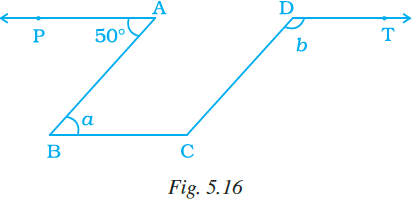(a) 60o, 120o (b) 50o,130o (c) 70o,110o (d) 80o,100o

Solution:-

(b) 50o,130o

We know that, ∠PAB = ∠ABC = 50o … [because interior alternate angles]

Given, AB || DC so consider it as parallelogram,

In a parallelogram, adjacent angles of the parallelogram are supplementary.

So, ∠ABC + ∠BCD = 180o

50o + ∠BCD = 180o

∠BCD = 180o – 50o

∠BCD = 130o

∠BCD = ∠CDT = 130o  … [because interior alternate angles]

Therefore, a = 50o and b = 130o

18. The difference of two complementary angles is 30o. Then, the angles are

(a) 60o, 30o (b) 70o, 40o (c) 20o, 50o (d) 105o, 75o

Solution:-

(a) 60o, 30o

When the sum of the measures of two angles is 90o, then the angles are called complementary angles.

So, 60o + 30o = 90o

As per the condition in the question, 60o – 30o = 30o

19. In Fig. 5.17, PQ || SR and SP || RQ. Then, angles a and b are respectively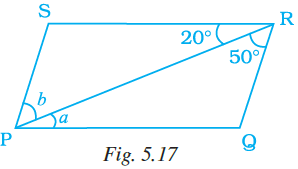(a) 20o, 50o (b) 50o, 20o (c) 30o, 50o (d) 45o, 35o

Solution:-

(a) 20o, 50o

∠QRP = ∠RPS = 50o  … [because interior alternate angles]

∠SRP = ∠RPQ = 20o … [because interior alternate angles]

Therefore, angle a = 20o and angle b = 50o

20. In Fig. 5.18, a and b are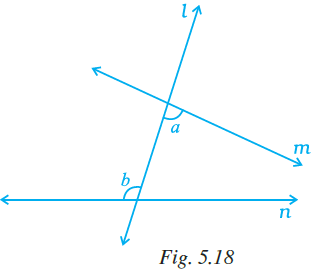(a) alternate exterior angles

(b) corresponding angles

(c) alternate interior angles

(d) vertically opposite angles

Solution:-

(c) alternate interior angles

21. If two supplementary angles are in the ratio 1: 2, then the bigger angle is

(a) 120o (b) 125o (c) 110o (d) 90o

Solution:-

(a) 120o

We know that, when the sum of the measures of two angles is 180o, then the angles are called supplementary angles.

Let us assume two angles be 1x and 2x.

1x + 2x = 180o

3x = 180o

x = 180o/3

x = 60o

Then the bigger angle is 2x = 2 × 60o = 120o

22. In Fig. 5.19, ∠ROS is a right angle and ∠POR and ∠QOS are in the ratio 1: 5. Then, ∠QOS measures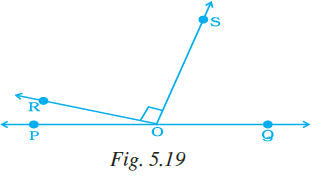(a) 150o (b) 75o (c) 45o (d) 60o

Solution:-

(b) 75o

The sum of all angles about a straight line given in the figure is equal to 180o.

Given, ∠ROS is a right angle = 90o

Let us assume ∠POR = x and ∠QOS = 5x.

Then, ∠POR + ∠ROS + ∠QOS = 180o

x + 90+ 5x = 180o

6x = 180o – 90o

6x = 90o

x = 90o/6

x = 15o

So, ∠QOS measures = 5x = 5 × 15o = 75o

23. Statements a and b are as given below:

a: If two lines intersect, then the vertically opposite angles are equal.

b: If a transversal intersects two other lines, then the sum of two interior angles on the same side of the transversal is 180o.

Then

(a) Both a and b are true (b) a is true and b is false

(c) a is false and b is true (d) both a and b are false

Solution:-

(b) a is true and b is false

24. For Fig. 5.20, statements p and q are given below:

p: a and b are forming a linear pair.

q: a and b are forming a pair of adjacent angles.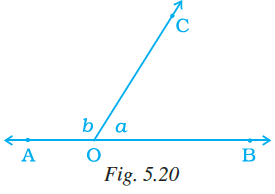Then,

(a) both p and q are true

(b) p is true and q is false

(c) p is false and q is true

(d) both p and q are false

Solution:-

(a) both p and q are true

25. In Fig. 5.21, ∠AOC and ∠ BOC form a pair of

(a) vertically opposite angles

(b) complementary angles

(c) alternate interior angles

(d) supplementary angles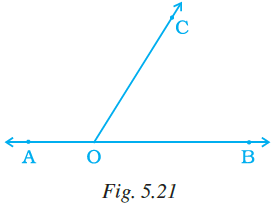Solution:-

(d) supplementary angles

26. In Fig. 5.22, the value of a is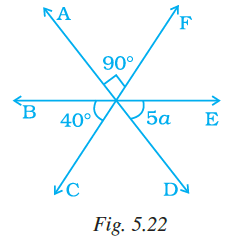(a) 20o (b) 15o

(c) 5o (d) 10o

Solution:-

(d) 10o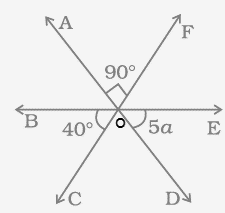∠AOF = ∠COD = 90o [because vertically opposite angles]

Sum of all angles about a straight line given in the figure are equal to 180o.

Then, ∠BOC + ∠COD + ∠DOE = 180o

40o + 90o + 5a = 180o

130o + 5a = 180o

5a = 180o – 130o

5a = 50o

a = 50/5

a = 10o

27. In Fig. 5.23, if QP || SR, the value of a is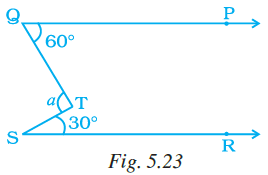(a) 40o (b) 30o (c) 90o (d) 80o

Solution:-

(c) 90o

To find out the value of ‘a’, draw a line XY, to cut at ‘a’.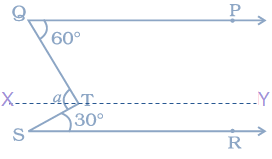So, XY || SR

∠XTS = ∠TSR = 30o … [because interior alternate angles]

∠PQT = ∠QTX = 60o … [because interior alternate angles]

Then, a = ∠XTS + ∠QTX

= 30o + 60o

= 90o

28. In which of the following figures, a and b are forming a pair of adjacent angles?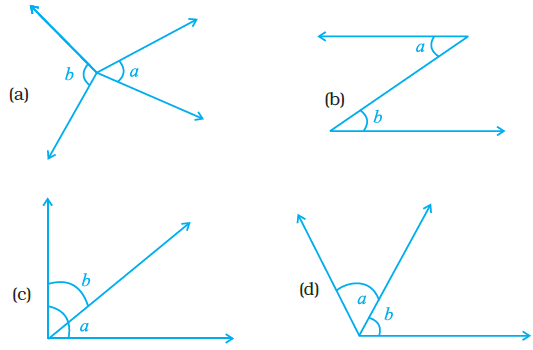Solution:-

In figure (d) a and b form a pair of adjacent angles.

29. In a pair of adjacent angles, (i) vertex is always common, (ii) one arm is always common, and (iii) uncommon arms are always opposite rays

Then

(a) All (i), (ii) and (iii) are true

(b) (iii) is false

(c) (i) is false but (ii) and (iii) are true

(d) (ii) is false

Solution:-

(b) (iii) is false

Two angles are called adjacent angles, if they have a common vertex and a common arm but no common interior points.

30. In Fig. 5.25, lines PQ and ST intersect at O. If ∠POR = 90° and x : y = 3 : 2, then z is equal to

(a) 126o (b) 144o (c) 136o (d) 154o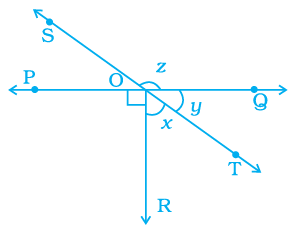Solution:-

(b) 144o

The sum of all angles about a straight line given in the figure is equal to 180o.

PQ is a straight line.

Then, ∠POR + ∠ROT + ∠TOQ = 180o

Given, x : y = 3 : 2

Let us assume x = 3a, y = 2a

90o + 3a + 2a = 180o

90o + 5a = 180o

5a = 180o – 90o

5a = 90o

a = 90/5

a = 18o

So, x = 3a = 3 × 18 = 54o

y = 2a = 2 × 18 = 36o

From the figure SOT is a straight line,

Then, z+ y = 180o

z + 36o = 180o

z = 180o – 36o

z = 144o

31. In Fig. 5.26, POQ is a line, then a is equal to

(a) 35o (b) 100o (c) 80o (d) 135o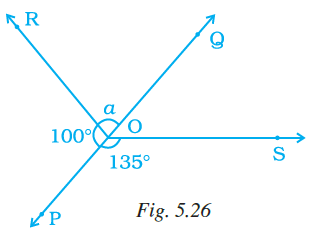Solution:-

(c) 80o

From the figure POQ is a straight line,

Then, 100 + a = 180o

a = 180o – 100

a= 80o

32. Vertically opposite angles are always

(a) supplementary (b) complementary

Solution:-

(d) equal

33. In Fig. 5.27, a = 40o. The value of b is

(a) 20o (b) 24o (c) 36o (d) 120o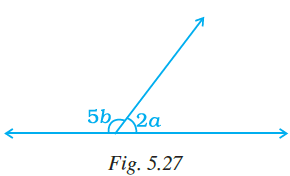Solution:-

(a) 20o

Given, a = 40o

Then, 2a = 2 × 40 = 80o

From the figure, angles formed on the straight line are equal to 180o,

Then, 5b + 2a = 180o

5b + 80o = 180o

5b= 180o – 80o

5b = 100o

b = 100/5

b = 20o

34. If an angle is 60o less than two times of its supplement, then the greater angle is

(a) 100o (b) 80o (c) 60o (d) 120o

Solution:-

(a) 100o

Let us assume the angle be P.

Then, its supplement is 180o – P

As per the condition in the question,

P = 2(180o – P) – 60o

P = 360o – 2P – 60o

P + 2P = 300o

3P = 300o

P = 300/3

P = 100o

So, its supplement is 180o – P = 180o – 100o = 80o

Therefore, the greater angle is 100o.

35. In Fig. 5.28, PQ || RS. If ∠1=(2a+b)o and ∠6=(3a–b)o, then the measure of ∠2 in terms of b is

(a) (2+b)o (b) (3–b)o (c) (108–b)o (d) (180–b)o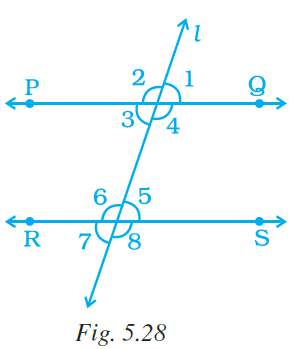Solution: –

(c) (108–b)o

From the question it is given that, ∠1 = (2a + b)o and ∠6 = (3a – b)o

Since ∠5 and ∠6 form a linear pair of angles

Then,

∠5 = (180-3a + b)o … [equation 1]

∠5 = ∠1 = (180-3a + b)o [Because Corresponding angles] …equation (2)

From equation (2) we get,

2a + b = 180-3a + b

5a = 180

a = 360

Since ∠1 and ∠2 forms a linear pair so

∠2 = 1800– 2a-b

Substituting the value of a

∠2 = 1800– 720 – b

∠2 = 1080– b

36. In Fig. 5.29, PQ||RS and a : b = 3 : 2. Then, f is equal to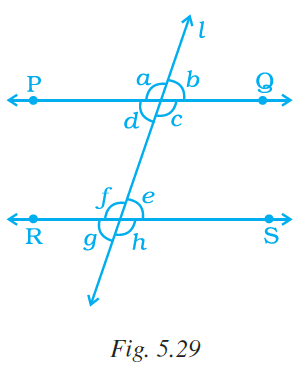(a) 36o (b) 108o (c) 72o (d) 144o

Solution: –

(b) 108o

From the figure, PQ||RS.

From the question it is given that, a: b = 3: 2

So, let us assume a = 3m and b = 2m

We know that, sum of angles on the straight line is equal to 180o

Then, ∠a + ∠b = 180o

3m + 2m = 180o

5m = 180o

m = 180o/5

m = 36o

So, a = 3m = 3 × 36o = 108o

b = 2m = 2 × 36o = 72

Therefore, ∠a = ∠f = 108o [because corresponding angles]

37. In Fig. 5.30, line l intersects two parallel lines PQ and RS. Then, which one of the following is not true?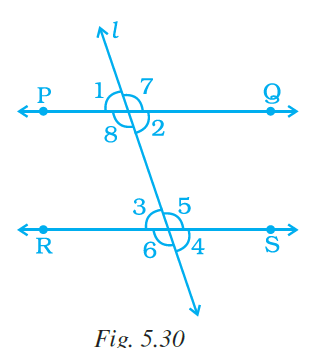(a) ∠1 = ∠3 (b) ∠2 = ∠4

(c) ∠6 = ∠7 (d) ∠4 = ∠8

Solution:-

(d) ∠4 = ∠8

Because, ∠4 ≠ ∠8

38. In Fig. 5.30, which one of the following is not true?(a) ∠1 + ∠5 = 180o

(b) ∠2 + ∠5 = 180o

(c) ∠3 + ∠8 = 180o

(d) ∠2 + ∠3 = 180o

Solution:-

(d) ∠2 + ∠3 = 180o

We know that, interior opposite angles are equal

∠2 = ∠3

39. In Fig. 5.30, which of the following is true?(a) ∠1 = ∠5 (b) ∠4 = ∠8 (c) ∠5 = ∠8 (d) ∠3 = ∠7

Solution:-

(c) ∠5 = ∠8

From the figure, PQ||RS

∠5 = ∠8 [interior alternate angles are equal]

40. In Fig. 5.31, PQ||ST. Then, the value of x + y is

(a) 125o (b) 135o (c) 145o (d) 120o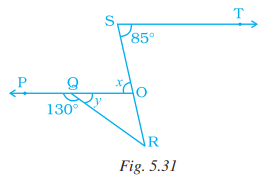Solution: –

(b) 135o

From the figure, PO is a straight line

We know that, sum of angles on the straight is equal to 180o.

Then,

y + ∠ PQR = 1800

y + 1300 = 1800

y = 50o

Then,

∠ QOS = ∠ TSO [Co-interior angle]

x = 850

x + y = 135

41. In Fig. 5.32, if PQ||RS and QR||TS, then the value a is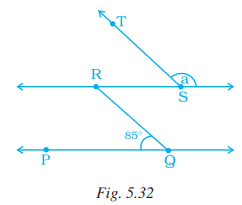(a) 95o (b) 90o (c) 85o (d) 75o

Solution:-

(a) 95o

We know that, corresponding angles are equal

So,

∠ RQP = ∠ TSR = 850 (Corresponding angles)

a + ∠ TSR = 1800

∠a = 95

In questions 42 to 56, fill in the blanks to make the statements true.

42. If sum of measures of two angles is 90o, then the angles are _________.

Solution:-

If sum of measures of two angles is 90o, then the angles are complementary.

43. If the sum of measures of two angles is 180o, then they are _________.

Solution:-

If the sum of measures of two angles is 180o, then they are supplementary.

44. A transversal intersects two or more than two lines at _________ points.

Solution:-

A transversal intersects two or more than two lines at distinct points.

If a transversal intersects two parallel lines, then (Q. 45 to 48).

45. The sum of interior angles on the same side of a transversal is

Solution:-

The sum of interior angles on the same side of a transversal is 180o.

46. Alternate interior angles have one common.

Solution:-

Alternate interior angles have one common arm.

47. Corresponding angles are on the side of the transversal.

Solution:-

Corresponding angles are on the same side of the transversal.

48. Alternate interior angles are on the side of the transversal.

Solution:-

Alternate interior angles are on the opposite side of the transversal

49. Two lines in a plane which do not meet at a point anywhere are called lines.

Solution:-

Two lines in a plane which do not meet at a point anywhere are called parallel lines.

50. Two angles forming a __________ pair are supplementary.

Solution:-

Two angles forming a linear pair are supplementary.

51. The supplement of an acute is always __________ angle.

Solution:-

The supplement of an acute is always an obtuse angle.

52. The supplement of a right angle is always _________ angle.

Solution:-

The supplement of a right angle is always right angle.

53. The supplement of an obtuse angle is always _________ angle.

Solution:-

The supplement of an obtuse angle is always an acute angle.

54. In a pair of complementary angles, each angle cannot be more than _________.

Solution:-

In a pair of complementary angles, each angle cannot be more than 90o.

55. An angle is 45o. Its complementary angle will be __________ .

Solution:-

An angle is 45o. Its complementary angle will be 45o.

56. An angle which is half of its supplement is of __________.

Solution:-

An angle which is half of its supplement is 60o.

Let us assume the angle is p, and the supplement is 2p

p + 2p = 1800

3p = 1800

p = 600